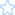#### Apache Rewrite解决问号匹配的写法2012/11/05 17:33ArthurXF

A地址：域名/ProductView.jsp?lClassID=200

B地址：域名/goods.php?id=3

. 任意一个单字符
[chars] 字符类: "chars"中的任意一个字符
[^chars] 字符类: 不在"chars"中的字符
text1|text2 选择: text1 或 text2

? 前面的字符出现 0 或 1 次
* 前面的字符出现 0 或 N 次(N > 0)
+ 前面的字符出现 1 或 N 次(N > 1

RewriteCond %{QUERY_STRING} ^lClassID=200\$
RewriteRule ^ProductView\.jsp\$ goods\.php\?id=3 [L]

RewriteEngine on
RewriteCond %{QUERY_STRING} ^id=(.+)\$
RewriteRule ^/abc\$ /def.php?sid=%1 [L]

RewriteRule 不会去匹配 ? 后面的字符串，需要用RewriteCond来匹配

Tags:

#### JavaScript：多维数组像ORDER BY一样实现多键值排序2012/11/05 13:28ArthurXF

JavaScript的数组排序函数 sort方法，默认是按照ASCII 字符顺序进行升序排列。

arrayobj.sort(sortfunction);

sort 方法将 Array 对象进行适当的排序；在执行过程中并不会创建新的 Array 对象。

• 负值，如果所传递的第一个参数比第二个参数小。
• 零，如果两个参数相等。
• 正值，如果第一个参数比第二个参数大。<script language=javascript>var myArray
= new Array();for(var i=0;i<10;i++ )...{myArray[i]
=new Array();myArray[i][
0]=Math.floor(Math.random()*10);myArray[i][
1]=Math.floor(Math.random()*10);myArray[i][
2]=Math.floor(Math.random()*10);myArray[i][
3]=Math.floor(Math.random()*10);myArray[i][
4]=Math.floor(Math.random()*10);myArray[i][
5]=Math.floor(Math.random()*10);myArray[i][
6]=Math.floor(Math.random()*10);myArray[i][
7]=Math.floor(Math.random()*10);myArray[i][
8]=Math.floor(Math.random()*10);}myArray.sort( function(x, y)
...{return (x==y)?((x==y)?(x-y):(x-y)):(x-y)}
);for(var i=0;i<myArray.length;i++ )...{document.write(myArray[i].join(
",") + "<br/>");}</script>function sortFunction(array) ...{return array.sort( function(x, y) ...{return (x==y)?(x.localeCompare(y)):(x.localeCompare(y))});}

Tags: